# How to Join Pandas DataFrames using Merge?

## How to Join Pandas DataFrames using Merge?

Joining and merging DataFrames is that the core process to start out out with data analysis and machine learning tasks. It’s one of the toolkits which each Data Analyst or Data Scientist should master because in most cases data comes from multiple sources and files. In this tutorial, you’ll how to join data frames in pandas using the merge technique. More specifically, we will practice the concatenation of DataFrames along row and column.

## Getting Started

The most widely used operation related to DataFrames is the merging operation. Two DataFrames might hold different kinds of information about the same entity and they may have some same columns, so we need to combine the two data frames in pandas for better reliability code. To join these DataFrames, pandas provides various functions like join(), concat(), merge(), etc. In this section, you will practice using the merge() function of pandas.

There are basically four methods of merging:

• inner join
• outer join
• right join
• left join

### Inner join

From the name itself, it is clear enough that the inner join keeps rows where the merge “on” value exists in both the left and right dataframes.Now let us create two dataframes and then try merging them using inner.

 `import` `numpy as np` `import` `pandas as pd` ` ` ` ` `left ``=` `pd.DataFrame({``'Sr.no'``: [``'1'``, ``'2'``, ``'3'``, ``'4'``, ``'5'``], ` `                    ``'Name'``: [``'Rashmi'``, ``'Arun'``, ``'John'``, ` `                             ``'Kshitu'``, ``'Bresha'``], ` `                    ``'Roll No'``: [``'1'``, ``'2'``, ``'3'``, ``'4'``, ``'5'``]}) ` ` ` `right ``=` `pd.DataFrame({``'Sr.no'``: [``'2'``, ``'4'``, ``'6'``, ``'7'``, ``'8'``], ` `                    ``'Gender'``: [``'F'``, ``'M'``, ``'M'``, ``'F'``, ``'F'``], ` `                    ``'Interest'``: [``'Writing'``, ``'Cricket'``, ``'Dancing'``,` `                                 ``'Chess'``, ``'Sleeping'``]}) ` `                         ` `# Merging the dataframes                     ` `pd.merge(left, right, how ``=``'inner'``, on ``=``'Sr.no'``) `

Output: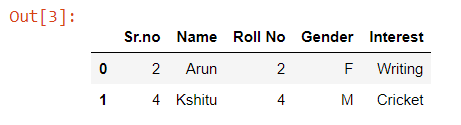### Outer join

An outer join returns all the rows from the left dataframe, all the rows from the right dataframe, and matches up rows where possible, with NaNs elsewhere. But if the dataframe is complete, then we get the same output.`import` `numpy as np` `import` `pandas as pd` ` ` ` ` `left ``=` `pd.DataFrame({``'Sr.no'``: [``'1'``, ``'2'``, ``'3'``, ``'4'``, ``'5'``], ` `                    ``'Name'``: [``'Rashmi'``, ``'Arun'``, ``'John'``,` `                             ``'Kshitu'``, ``'Bresha'``], ` `                    ``'Roll No'``: [``'1'``, ``'2'``, ``'3'``, ``'4'``, ``'5'``]}) ` ` ` `right ``=` `pd.DataFrame({``'Sr.no'``: [``'2'``, ``'4'``, ``'6'``, ``'7'``, ``'8'``], ` `                    ``'Gender'``: [``'F'``, ``'M'``, ``'M'``, ``'F'``, ``'F'``], ` `                    ``'Interest'``: [``'Writing'``, ``'Cricket'``, ``'Dancing'``, ` `                                 ``'Chess'``, ``'Sleeping'``]}) ` `                         ` `# Merging the dataframes                     ` `pd.merge(left, right, how ``=``'outer'``, on ``=``'Sr.no'``)`

Output: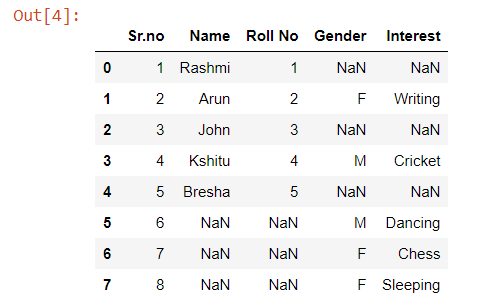### Left join

With a left join, all the records from the first dataframe will be displayed, irrespective of whether the keys in the first dataframe can be found in the second dataframe. Whereas, for the second dataframe, only the records with the keys in the second dataframe that can be found in the first dataframe will be displayed.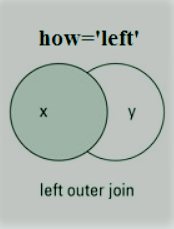`import` `numpy as np` `import` `pandas as pd` ` ` ` ` `left ``=` `pd.DataFrame({``'Sr.no'``: [``'1'``, ``'2'``, ``'3'``, ``'4'``, ``'5'``], ` `                    ``'Name'``: [``'Rashmi'``, ``'Arun'``, ``'John'``, ` `                             ``'Kshitu'``, ``'Bresha'``], ` `                    ``'Roll No'``: [``'1'``, ``'2'``, ``'3'``, ``'4'``, ``'5'``]}) ` ` ` `right ``=` `pd.DataFrame({``'Sr.no'``: [``'2'``, ``'4'``, ``'6'``, ``'7'``, ``'8'``], ` `                    ``'Gender'``: [``'F'``, ``'M'``, ``'M'``, ``'F'``, ``'F'``], ` `                    ``'Interest'``: [``'Writing'``, ``'Cricket'``, ` `                                 ``'Dancing'``, ``'Chess'``, ` `                                 ``'Sleeping'``]}) ` `                         ` `# Merging the dataframes                     ` `pd.merge(left, right, how ``=``'left'``, on ``=``'Sr.no'``)`

Output: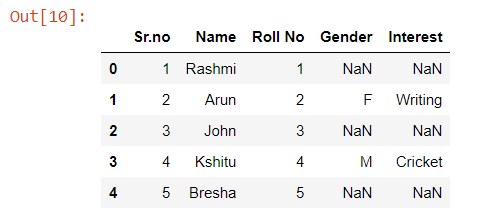Note the Output Carefully.

### Right join

For a right join, all the records from the second dataframe will be displayed. However, only the records with the keys in the first dataframe that can be found in the second dataframe will be displayed.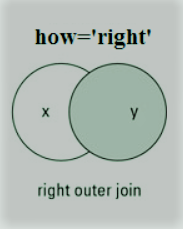`import` `numpy as np` `import` `pandas as pd` ` ` ` ` `left ``=` `pd.DataFrame({``'Sr.no'``: [``'1'``, ``'2'``, ``'3'``, ``'4'``, ``'5'``], ` `                    ``'Name'``: [``'Rashmi'``, ``'Arun'``, ``'John'``,` `                             ``'Kshitu'``, ``'Bresha'``], ` `                    ``'Roll No'``: [``'1'``, ``'2'``, ``'3'``, ``'4'``, ``'5'``]}) ` ` ` `right ``=` `pd.DataFrame({``'Sr.no'``: [``'2'``, ``'4'``, ``'6'``, ``'7'``, ``'8'``], ` `                    ``'Gender'``: [``'F'``, ``'M'``, ``'M'``, ``'F'``, ``'F'``], ` `                    ``'Interest'``: [``'Writing'``, ``'Cricket'``, ``'Dancing'``, ` `                                 ``'Chess'``, ``'Sleeping'``]}) ` `                         ` `# Merging the dataframes                     ` `pd.merge(left, right, how ``=``'right'``, on ``=``'Sr.no'``) `

Output: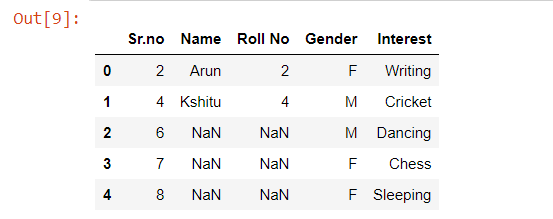Last Updated on October 23, 2021 by admin

## How to Find & Drop duplicate columns in a Pandas DataFrame?How to Find & Drop duplicate columns in a Pandas DataFrame?

How to Find & Drop duplicate columns in a Pandas DataFrame? Let’s discuss How to

## Pandas DataFrame.to_stringPandas DataFrame.to_string

Pandas DataFrame.to_string Pandas DataFrame is a two-dimensional size-mutable, potentially heterogeneous tabular data structure with labeled

## How to append a list as a row to a Pandas DataFrame in Python?How to append a list as a row to a Pandas DataFrame in Python?

How to append a list as a row to a Pandas DataFrame in Python? In

## Python | Pandas dataframe.replace()Python | Pandas dataframe.replace()

Python –  Pandas dataframe.replace() Pandas dataframe.replace() function is used to replace a string, regex, list,

## Get a list of a specified column of a Pandas DataFrameGet a list of a specified column of a Pandas DataFrame

Get a list of a specified column of a Pandas DataFrame In this article, we

## pandas.crosstab() function in Pythonpandas.crosstab() function in Python

pandas.crosstab() function in Python This method is used to compute a simple cross-tabulation of two

## Python | Pandas dataframe.reindex()Python | Pandas dataframe.reindex()

Python | Pandas dataframe.reindex() Python is a great language for doing data analysis, primarily because

## Pandas dataframe.equals()Pandas dataframe.equals()

Python Pandas dataframe.equals() Python is a great language for doing data analysis, primarily because of